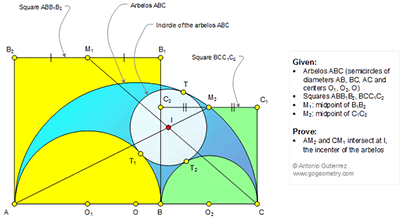## Saturday, December 31, 2016

### Geometry Problem 1301: Arbelos, Semicircles, Diameters, Circle, Incircle, Incenter, Square, Midpoint, Concurrency

Geometry Problem. Post your solution in the comment box below.
Level: Mathematics Education, High School, Honors Geometry, College.

Details: Click on the figure below.1.Problem 1301
Let K medium the arc AB and L medium the arc BC, then K is medium M1O1 and L is medium M2O2.Draw IH perpendicular in AC ,
intersecting the circle (Ι,ΙΤ) in P.So from problem 1298 is IP=PH.
But IP/PH=M1K/KO1=M2L/LO2 =1 so the points M1,I and C are collinear and points A,I and M2 are collinear.
APOSTOLIS MANOLOUDIS KORYDALLOS PIRAEUS GREECE

1.To Apostolis

Referring to last line of your solution " But IP/PH=M1K/KO1=M2L/LO2 =1 so the points M1,I and C are collinear and points A,I and M2 are collinear"
In my opinion , we need to show that points C, P and K are collinear before conclude that M1, I and C are collinear.

2.Yes to peter.
The points C,P and T1 are collinear from problem 1300 , <BT1C+<AT1B+<KT2A=45+90+45=180
so the points K,T1,P and C are collinear. Thenks

2.Relation between three inradius (for all three circles inscribed in arbelos)
Ri=[R1R2(R1+R2)]/(R1²+R1R2+R2²)

3.PN perpendicular to AC, P intersection CM1 and AM2, tr PNA ~ tr M2O2A
and tr PNC ~ tr M1O1C + Theorem pythagore for tr O1IN and O2IN
then just to prove PN = 2Ri